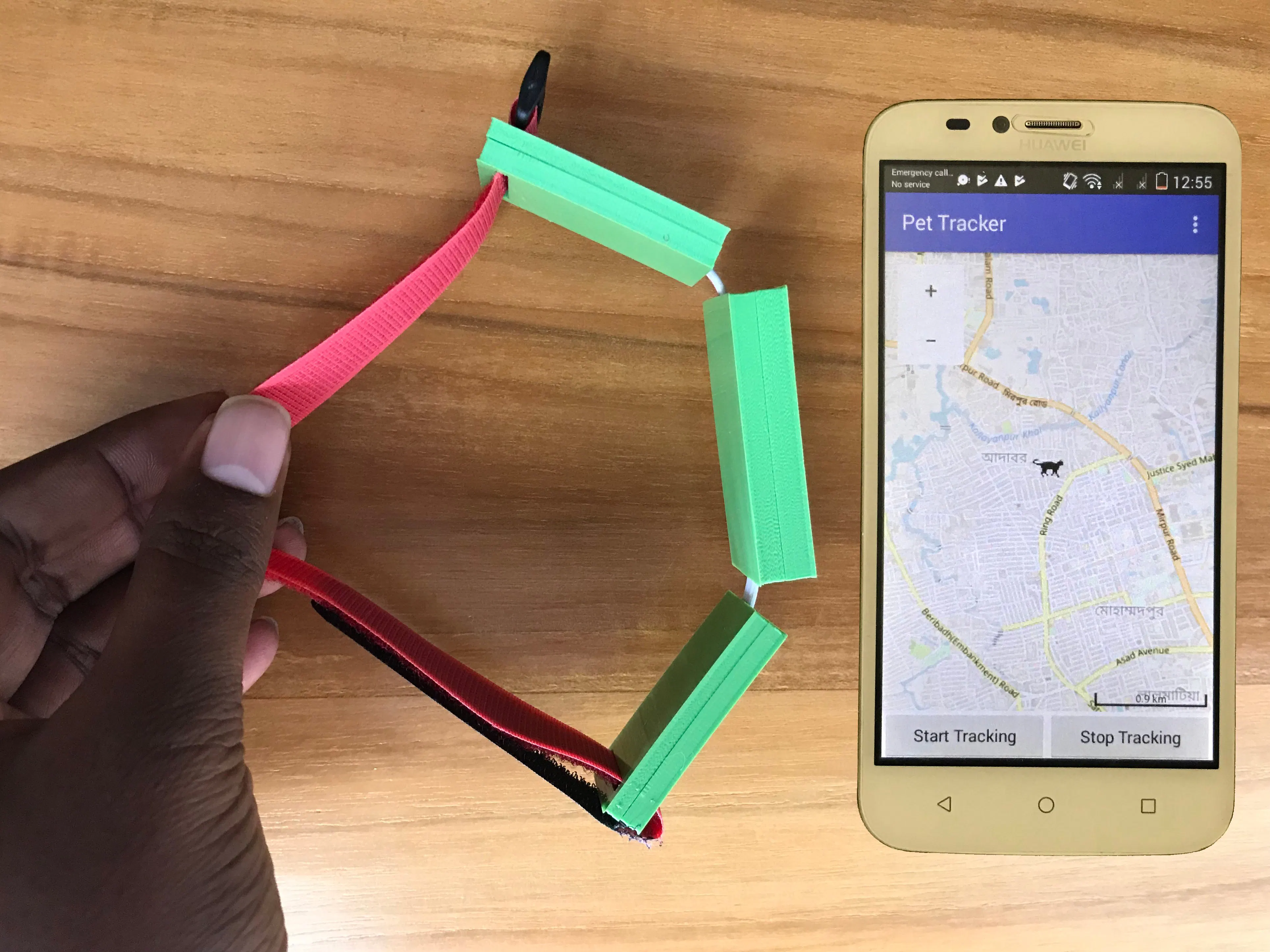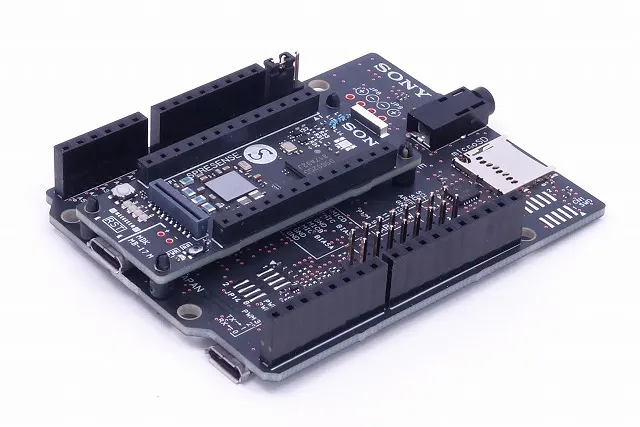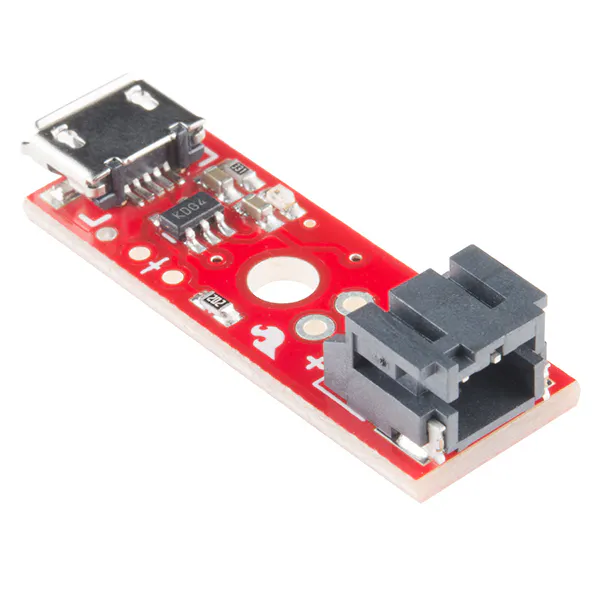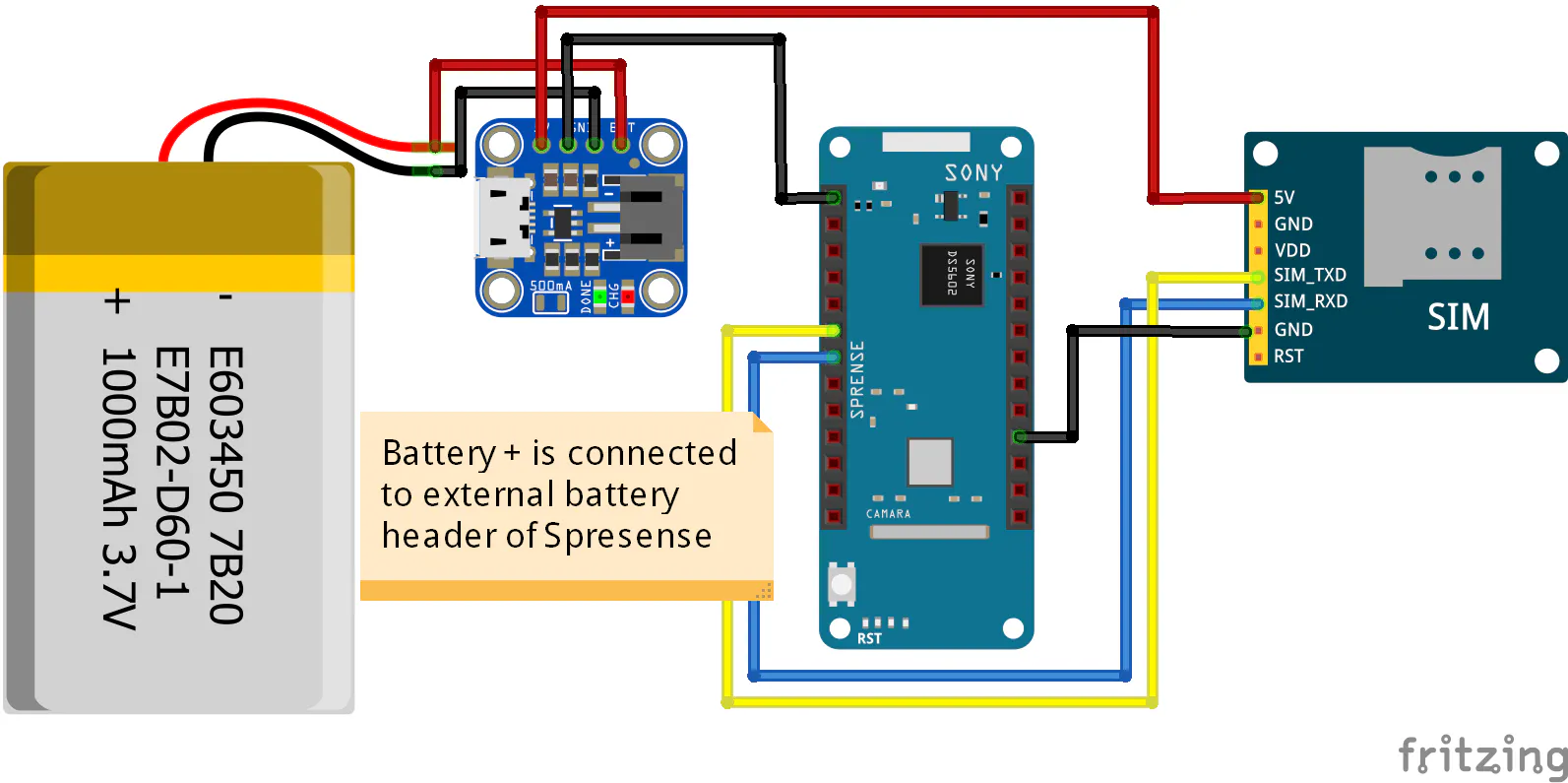A pet tracker based on the Sony Spresense development board.## Things used in this project

### Hardware componentsSony Spresense boards (main & extension)
×1
 SIM800 GPRS Module
×1
×1SparkFun LiPo Charger Basic - Micro-USB
×1

### Software apps and online servicesArduino IDE

### Hand tools and fabrication machines3D Printer (generic)

## Schematics

### Schematic Diagram

Designed in fritzing## Code

### Pet Locator Arduino Sketch

Arduino
SIM800 was used for GPRS communication and http is implemented using AT command.
```/* include the GNSS library */
#include <GNSS.h>
#include<math.h>

#include <SoftwareSerial.h>
//software serial is for connecting GPRS module
SoftwareSerial GPRS(26, 27); // RX, TX

#define RESTART_CYCLE       (60 * 5)
static SpGnss Gnss;                   /**< SpGnss object */

//Ubidots credentials
#define TOKEN "A1E-wy1sLSSOhc2uhB1L31oL7hL1f8v1DE"  // Remplace it with your token

#define ID1 "5c6b52fe5916361009c83050" // variable ID for flag
#define ID2 "5c6ad8eec03f970ee849e608" // for variable lat
#define ID3 "5c6ad8d8c03f970e872bc451" // for variable long

char latoutput;
char longoutput;

void setup() {

/* For serial debugging */
Serial.begin(115200);
// Serial com for communicating with GPRS Modulr
GPRS.begin(19200);
/* Wait HW initialization done. */
sleep(3);
/* Set Debug mode to Info */
Gnss.setDebugMode(PrintInfo);

int result;

/* Activate GNSS device */
result = Gnss.begin();

if (result == 0)
{
//configuring gprs type
Gnss.select(GPS);
Gnss.select(GLONASS);
Gnss.select(QZ_L1CA);
/* Start positioning */
result = Gnss.start(COLD_START);
if (result != 0)
{
exit(0);
}

}

}

/**
* @brief %Print position information.
*/
static void print_pos(SpNavData *pNavData)
{
if (pNavData->posFixMode == FixInvalid)
{
Serial.print("No-Fix, ");
}
else
{
Serial.print("Fix, ");
}
if (pNavData->posDataExist == 0)
{
Serial.print("No Position");
}
else
{
float longi = pNavData->longitude;
float lati = pNavData->latitude;
ftoa(lati,latoutput,6);
ftoa(longi,longoutput,6);
Serial.print("Lat=");
Serial.print(latoutput);
Serial.print(", Lon=");
Serial.println(longoutput);
send_data_to_ubidot(latoutput, ID2); //send latitude data to ubidots
send_data_to_ubidot(longoutput, ID3);//send longitude data to ubidots
}
Serial.println("");
}

int sleep_flag=0;
void loop()
{
static int LoopCount = 0;
static int LastPrintMin = 0;
//get user data from ubidots either hw wants to trac or not
float flag = (int)get_data_from_ubidots(ID1);
//flag = 1 for tracking
if(flag==1&&sleep_flag==0){
SleepOut(); //wake up gps
sleep_flag=1;
}
else if(flag==0&&sleep_flag==1){
SleepIn(); //sleep gps to save power
sleep_flag=0;
}
/* Check update. */
if(sleep_flag==1){
if (Gnss.waitUpdate(-1))
{
/* Get NaviData. */
SpNavData NavData;
Gnss.getNavData(&NavData);

/* Print position information. */
print_pos(&NavData);
}
/* Check loop count. */
LoopCount++;
if (LoopCount >= RESTART_CYCLE)
{
int error_flag = 0;

/* Restart GNSS. */
if (Gnss.stop() != 0)
{
Serial.println("Gnss stop error!!");
error_flag = 1;
}
else if (Gnss.end() != 0)
{
Serial.println("Gnss end error!!");
error_flag = 1;
}
else
{
Serial.println("Gnss stop OK.");
}

if (Gnss.begin() != 0)
{
Serial.println("Gnss begin error!!");
error_flag = 1;
}
else if (Gnss.start(HOT_START) != 0)
{
Serial.println("Gnss start error!!");
error_flag = 1;
}
else
{
Serial.println("Gnss restart OK.");
}

LoopCount = 0;

/* Set error LED. */
if (error_flag == 1)
{
exit(0);
}
}

}

}
//go to sleep mode and save power
static void SleepIn(void)
{
Gnss.stop();
Gnss.end();
}

/**
* @brief Go to Active mode.
*/
static void SleepOut(void)
{
Gnss.begin();
Gnss.start(HOT_START);
}
// reverses a string 'str' of length 'len'
void reverse(char *str, int len)
{
int i=0, j=len-1, temp;
while (i<j)
{
temp = str[i];
str[i] = str[j];
str[j] = temp;
i++; j--;
}
}

// Converts a given integer x to string str[].  d is the number
// of digits required in output. If d is more than the number
// of digits in x, then 0s are added at the beginning.
int intToStr(int x, char str[], int d)
{
int i = 0;
while (x)
{
str[i++] = (x%10) + '0';
x = x/10;
}

// If number of digits required is more, then
// add 0s at the beginning
while (i < d)
str[i++] = '0';

reverse(str, i);
str[i] = '\0';
return i;
}

// Converts a floating point number to string.
void ftoa(float n, char *res, int afterpoint)
{
// Extract integer part
int ipart = (int)n;

// Extract floating part
float fpart = n - (float)ipart;

// convert integer part to string
int i = intToStr(ipart, res, 0);

// check for display option after point
if (afterpoint != 0)
{
res[i] = '.';  // add dot

// Get the value of fraction part upto given no.
// of points after dot. The third parameter is needed
// to handle cases like 233.007
fpart = fpart * pow(10, afterpoint);

intToStr((int)fpart, res + i + 1, afterpoint);
}
}

void httpInit(){
GPRS.println("AT"); /* Check Communication */
delay(200);
/* Configure bearer profile 1 */
GPRS.println("AT+SAPBR=3,1,\"CONTYPE\",\"Serial\"");  /* Connection type GPRS */
delay(2000);
GPRS.println("AT+SAPBR=3,1,\"APN\",\"gpinternet\"");  /* APN of the provider */
delay(3000);
GPRS.println("AT+SAPBR=1,1"); /* Open GPRS context */
delay(3000);
GPRS.println("AT+SAPBR=2,1"); /* Query the GPRS context */
delay(3000);
Serial.println("AT+HTTPINIT");  /* Initialize HTTP service */
delay(2000);
}

void send_data_to_ubidot(char* data, char* id){
httpInit();
GPRS.print(F("AT+HTTPPARA=\"URL\",\"things.ubidots.com/api/v1.6/variables/"));
GPRS.print(id);
GPRS.print(F("/values?token="));
GPRS.print(TOKEN);
GPRS.println("\"");
delay(4000);
GPRS.println(F("AT+HTTPPARA=\"CONTENT\",\"application/json\""));
delay(4000);
GPRS.print(F("AT+HTTPDATA="));
GPRS.print(strlen(data));
GPRS.print(F(","));
GPRS.println(120000);
delay(2000);
GPRS.write(data, strlen(data));
GPRS.println(F("AT+HTTPACTION=1"));  // HTTPACTION=1 is a POST method
delay(6000);
Serial.println("AT+HTTPTERM");  /* Terminate HTTP service */
delay(3000);
Serial.println("AT+SAPBR=0,1"); /* Close Serial context */
delay(3000);
}

float get_data_from_ubidots(char* id){
float num;
String raw;
flushInput();
httpTerm();
httpInit();
GPRS.print(F("AT+HTTPPARA=\"URL\",\"things.ubidots.com/api/v1.6/variables/"));
GPRS.print(id);
GPRS.print(F("/values?token="));
GPRS.print(TOKEN); //&page_size=1
GPRS.println(F("&page_size=1\""));
delay(1000);
GPRS.println(F("AT+HTTPACTION=0"));  //HTTPACTION=0 is GET method
delay(4000);
int bodyPosinit =9+ raw.indexOf("\"value\":");
int bodyPosend = raw.indexOf(", \"timestamp\"");
GPRS.println(F("AT+HTTPTERM"));
delay(1000);
return num;
}

while (timeout--) {
break;
}
while(GPRS.available()) {
if (c == '\r') continue;
if (c == 0xA) {
if (replyidx == 0)   // the first 0x0A is ignored
continue;
}
}

if (timeout == 0) {
break;
}
delay(1);
}

while(GPRS.available()){
}
}

void flushInput() {
// Read all available serial input to flush pending data.
uint16_t timeoutloop = 0;
while (timeoutloop++ < 40) {
while(Serial.available()) {
timeoutloop = 0;  // If char was received reset the timer
}
delay(1);
}
}

bool httpTerm(){
GPRS.println(F("AT+HTTPTERM"));
return false;
}
return true;
}
```

## Credits

### Md. Khairul Alam

43 projects • 401 followers
Engineer, developer, maker & hacker. Currently working as a faculty at the University of Asia Pacific, Dhaka, Bangladesh.